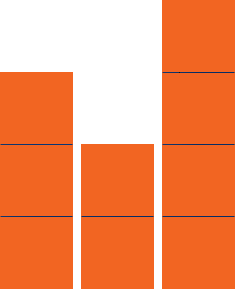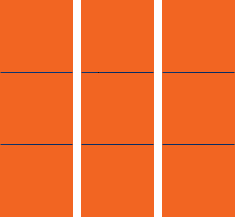SEARCH HOMEMath Central Quandaries & QueriesQuestion from Tammy, a parent: i need help for my son he is given 3 stacks of cubes. the 1rst stack has 3 the 2nd stack has 2 the 3rd stack has 4 the question is draw the stacks of cubes so they are arranged correctly to find the mean. what is the mean of the data?Hi Tammy,What the teacher wants your son to do is to see that if you move one block from the 4 block stack to the 2 block stack all the stacks will have the same number of blocks.Thus the mean or average number of blocks in a stack is 3.

I hope this helps,
PennyMath Central is supported by the University of Regina and The Pacific Institute for the Mathematical Sciences.